# Unknown amount of money

Damian and Denis split an unknown amount in the ratio of 5:4. Damian got six euros more than Denis. Calculate an unknown amount. Determine how much money got Damian and how Denis.

Correct result:

a =  30
b =  24
x =  54

#### Solution:

4a=5b
a=6+b
x=a+b

4•a=5•b
a=6+b
x=a+b

4a-5b = 0
a-b = 6
a+b-x = 0

a = 30
b = 24
x = 54

Our linear equations calculator calculates it.We would be pleased if you find an error in the word problem, spelling mistakes, or inaccuracies and send it to us. Thank you!Tips to related online calculators
Check out our ratio calculator.
Do you have a system of equations and looking for calculator system of linear equations?

## Next similar math problems:

• Money split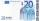Anton and Ferko got 2,500 euros together. The amount was split in 1:4 ratio. How much did each get?
• Dividing moneyImrich, Daniel and Dezider shared an unknown amount in the ratio 1:2:4, where Dezider received 750 euros more than Imrich and Daniel got half as much as Dezider. Determine an unknown amount of money and determine the amounth that got Imrich, Daniel and De
• CoinsDenis and Zdeno together have 97 coins. If Denis had 4 coins less than he has now, the number of the coins would be in the ratio 14: 17. Determine the number of coins owned by Denis and Zdeno.
• Trio ratioHans, Alena and Thomas have a total of 740 USD. Hans and Alena split in the ratio 5: 6 and Alena and Thomas in the ratio 4: 5. How much will everyone get?
• Division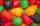Three siblings Helena, Oliver and George split the bag with candies on merit in the ratio 6:1:4. How many candies should each get if in bag were 88?
• Numbers at ratio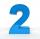The two numbers are in a ratio 3:2. If we each increase by 5 would be at a ratio of 4:3. What is the sum of original numbers?
• RepairingThree employees earned a total of € 469 for repairing the equipment. They split so that the first got 20% more than the second, and the third 15% more than the second. How many euros did everyone get?
• Price saleoffShoes standing y euros. At first they were discounted by 12% and then 50% of the new amount. After this double cheapening the cost was exactly 22 euros. Determine the original price of shoes.
• Money duoJulius and Mark have together 45 euros. Mark has 50% more money than Julius. Determine the amount of money that have Mark and Julius.
• Moneys in triple ratioMilan, John and Lili have a total 344 euros. Their amounts are in the ratio 1:2:5. Determine how much each of them has?
• Euros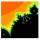Michal, Peter, John and Lenka got together 2,400 euros. They share an amount in ratio 2:6:4:3. How many got each of them?
• Revenue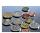Mr. Robert and Mr. Norbert get together € 1400. The revenue split in the ratio of 4:3, depending upon each work. How many got each of them?
• Ratio of two unknown numbersTwo numbers are given. Their sum is 30. We calculate one-sixth of a larger number and add to both numbers. So we get new numbers whose ratio is 5:7. Which two numbers were given?
• Function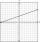For linear function f(x) = ax + ​​b ‬ is f(14)=179; f(15)=154. Calculate m, if f(m) = 2019 .
• SummandsWe want to split the number 110 into three summands so that the first and the second summand are in the ratio 4: 5, and the third with the first are in ratio 7: 3. Calculate the smallest of the summands.
• Isosceles trapezoidCalculate the content of an isosceles trapezoid whose bases are at ratio 5:3, the arm is 6cm long and it is 4cm high.
• Store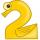Peter paid in store 3 euros more than half the amount that was on arrival to the store. When he leave shop he left 10 euros. How many euros he had upon arrival to the store?